## Excel SUMPRODUCT function

In Excel, the SUMPRODUCT function can be used to multiply two or more columns or arrays together, and then get the sum of products. In fact, the SUMPRODUCT is a useful function which can help to count or sum cell values with multiple criteria like the COUNTIFS or SUMIFS function. This article will introduce the function syntax and some examples for this SUMPRODUCT function.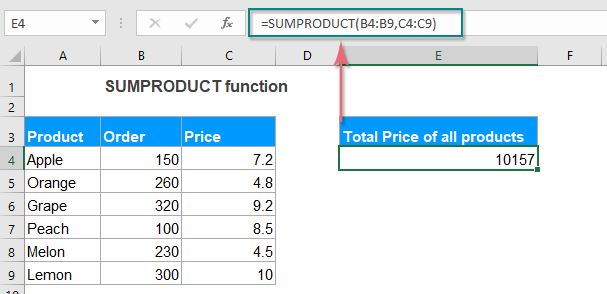#### Syntax:

The syntax for the SUMPRODUCT function in Excel is:

=SUMPRODUCT (array1, [array2], ...)

#### Arguments:

• array1: Required. The first array or range of cells you want to multiply and then add.
• array2: Optional. The second array or range of cells you want to multiply and then add.

Notes:

• 1. If there are non-numeric values in your range, the SUMPRODUCT treats them as zeros.
• 2. If the arrays or ranges have different number of rows and columns, the SUMPRODUCT function will return a #VALUE! error.
• 3. If there are logical tests in the arrays, they will create TRUE and FALSE values. In most cases, you'd need to convert them to 1 and 0 by using the double unary operator (--).
• 4. SUMPRODUCT function can handle up to 255 arguments in Excel 2007 and later versions, and only 30 arguments in earlier Excel versions.
• 5. SUMPRODUCT does not support wildcard characters.

#### Return:

Return the result of multiplied and summed arrays.

#### Example 1: Basic use of SUMPRODUCT function

The basic use of the SUMPRODUCT is to multiply two columns and then add them up, for example, you have the following screenshot data, now you want to multiply the order and the unit price, and then sum all the multiply results together to get the total price.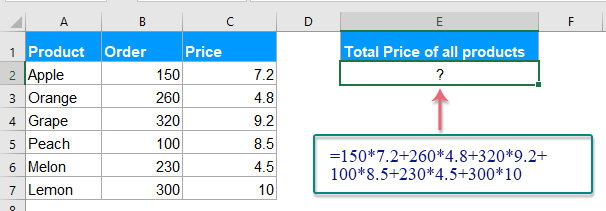=SUMPRODUCT(B2:B7,C2:C7)

Explanation: This formula SUMPRODUCT(B2:B7,C2:C7) = B2*C2+B3*C3+B4*C4+B5*C5+B6*C6+B7*C7.

And then, press Enter key, the cells in column B is multiplied by its corresponding cells in the same row of column C, and the results are added up. See screenshot: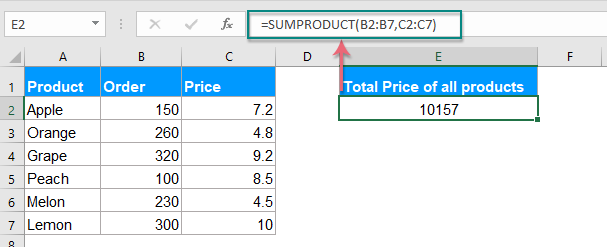#### Example 2: Sum cells with multiple criteria with SUMPRODUCT function

Except for the SUMIFS function to sum cell values based on multiple conditions, the SUMPRODUCT function also can solve this job quickly and easily.

##### Case 1: Sum cell values if contains specific text in another column

Supposing, you have a table of data as below screenshot shown, now, you want to calculate the total price which the product is KTE and the name is David, how could you do by using the SUMPRODUCT formula in Excel?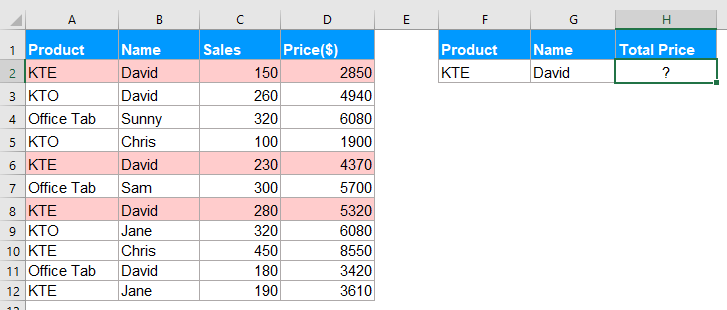1. Please enter or copy any one of the below formulas into a blank cell:

=SUMPRODUCT(--(A2:A12="KTE"), --(B2:B12="David"), D2:D12)
=SUMPRODUCT((A2:A12="KTE")*(B2:B12="David")*D2:D12)
• Tips: In the above formula:
• A2:A12="KTE": is the first criteria_range and criteria you want to sum based on.
• B2:B12="David": is the second criteria_range and criteria you want to sum based on.
• D2:D12: is the sum range that you want to sum the cell values.

2. Then, press Enter key to get the result as you need: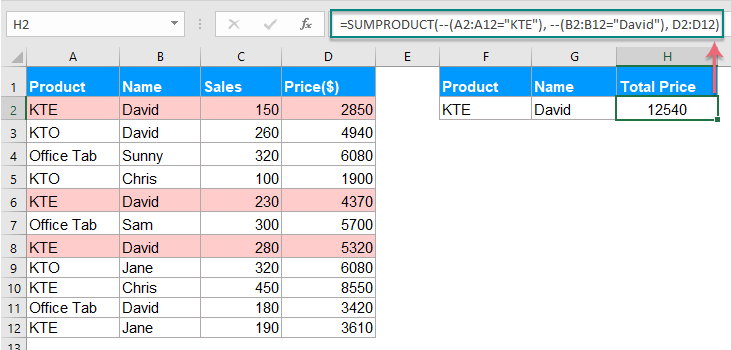##### Case2: Sum cells with multiple criteria with OR logic

To sum cells based on multiple criteria with OR logic, you should use the plus symbol (+) to join the multiple conditions together within the SUMPRODUCT function.

For example, I want to sum the total price of the product KTE and KTO in column A as below screenshot shown: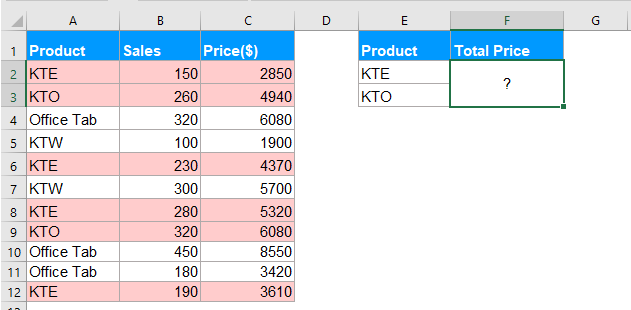Please apply the following formula into a blank cell:

=SUMPRODUCT((A2:A12="KTE")+(A2:A12="KTO"), C2:C12)

And then, press Enter key, the total price of the product KTE and KTO have been calculated, see screenshot: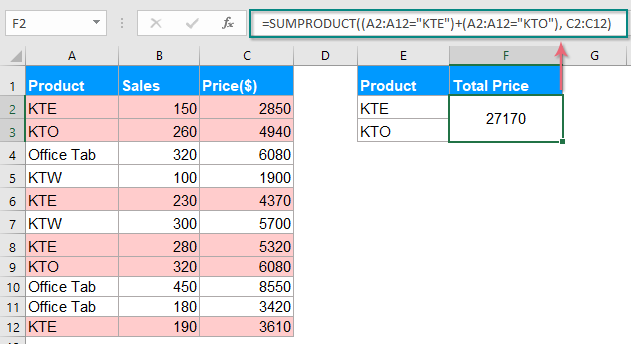##### Case3: Sum cells with multiple criteria with OR and AND logic

In some situations, you may need to sum cells with both OR and AND logic at the same time. The SUMPRODUCE function also can solve this job with ease.

Asterisk (*) is used as the AND operator.

Plus symbol (+) is used as the OR operator.

Let’s see an example, for summing the total price which product is KTE and KTO when the sale is greater than 200.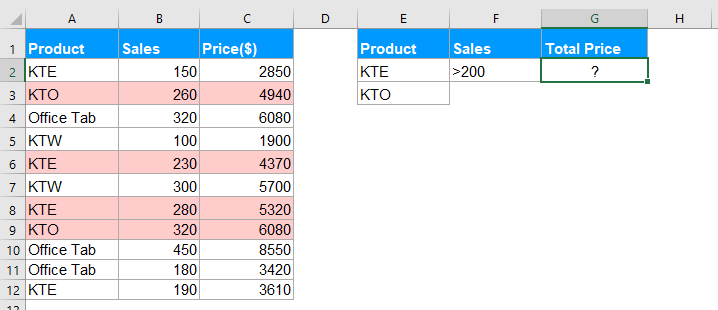You should copy or enter the below formula:

=SUMPRODUCT((B2:B12>200)*((A2:A12="KTE")+(A2:A12="KTO"))*C2:C12)

Then press Enter key to get the result as you need: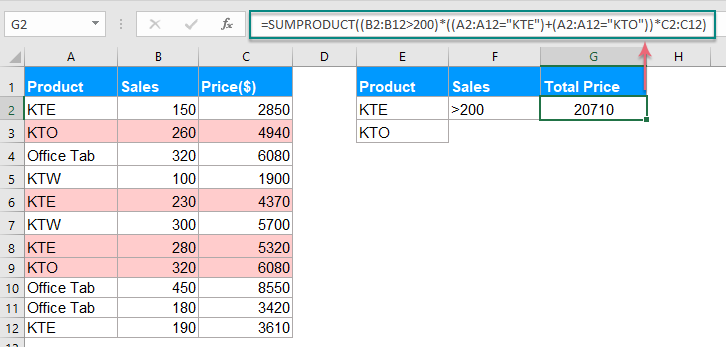#### Example 3: Count cells with multiple criteria with SUMPRODUCT function

Generally, this SUMPRODUCT function also can help us to count the cells with multiple criteria in Excel. As same as the sum values, you just need to remove the sum_range argument from the formula.

##### Case1: Count cells with multiple criteria with AND logic

Here, I want to count the cells that product is KTE and the sale is greater than 200. So, please use any one of the below formulas:

=SUMPRODUCT(--(A2:A12="KTE"), --(B2:B12>200))
=SUMPRODUCT((A2:A12="KTE")*(B2:B12>200))
• Tips: In the above formula:
• A2:A12="KTE": is the first criteria_range and criteria you want to count based on.
• B2:B12>200: is the second criteria_range and criteria you want to count based on.

And then, press Enter key to get the number of the result: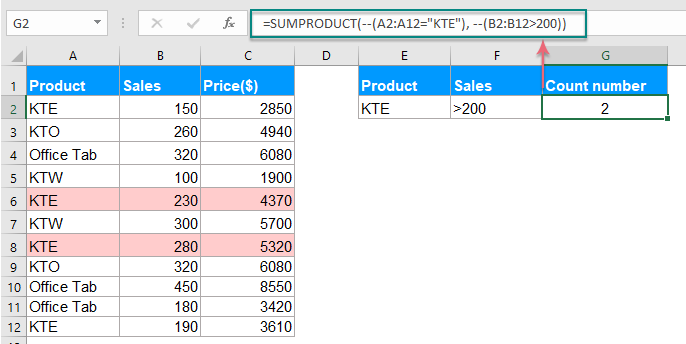##### Case2: Count cells with multiple criteria with OR logic

For counting the number of products KTE and KTO in column A, please apply this formula:

=SUMPRODUCT((A2:A12="KTE")+(A2:A12="KTO"))

And then, press Enter key to get the number of the product KTE and KTO. See screenshot: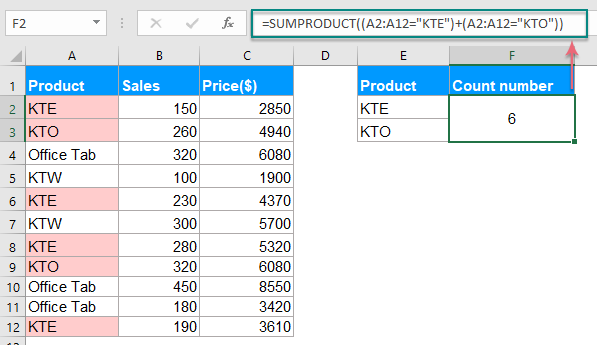##### Case3: Count cells with multiple criteria with OR and AND logic

To count the number of products KTE and KTO which sale is greater than 200, you should apply the following formula:

=SUMPRODUCT((B2:B12>200)*((A2:A12="KTE")+(A2:A12="KTO")))

Then, press Enter key to get the number of result you need: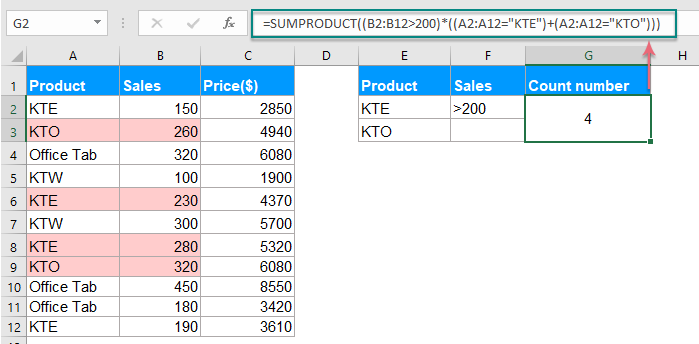#### Example 4: Calculate the weighted average with SUMPRODUCT function

Normally, we can calculate the average of range of cells quickly and easily. But, if you want to calculate the average of a list of values with different levels of relevance, it means to calculate the weighted average. There is no direct function for getting it in Excel. But, the SUMPRODUCT function can help you to deal with it.

For example, I have a table of grade statistics for a student, each task takes the different weight as below screenshot shown.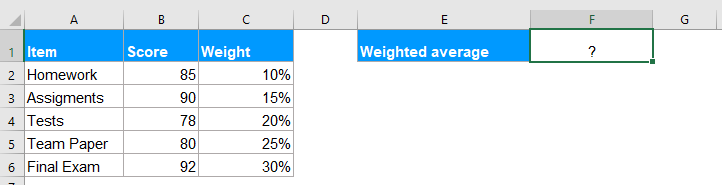To calculate the weighted average, please use the following formula:

=SUMPRODUCT(B2:B6, C2:C6) / SUM(C2:C6)

Explanation: This formula: SUMPRODUCT(B2:B6, C2:C6) / SUM(C2:C6) = (B2*C2+B3*C3+B4*C4+B5*C5+B6*C6)/(C2+C3+C4+C5+C6)

And then, press Enter key, the weighted average has been calculated at once, see screenshot: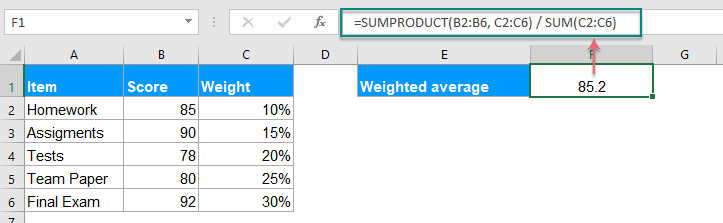#### More articles with SUMPRODUCT function:

• Countif A Specific Value Across Multiple Worksheets
• Supposing, I have multiple worksheets which contain the following data, and now, I want to get the number of occurrence of a specific value “Excel” from theses worksheets. How could I count specific values across multiple worksheets?
• Calculate Weighted Average In Excel
• For example, you have a shopping list with prices, weights, and amounts. You can easily calculate average price with the AVERAGE function in Excel. But what if weighted average price? In this article, I will introduce a method to calculate the weighted average, as well as a method to calculate weighted average if meeting specific criteria in Excel.

### The Best Office Productivity Tools

#### Kutools for Excel - Helps You To Stand Out From Crowd

 Popular Features: Find/Highlight/Identify Duplicates  |  Delete Blank Rows  |  Combine Columns or Cells without Losing Data  |  Round without Formula ... Super VLookup: Multiple Criteria  |  Multiple Value   |  Across Multi-Sheets  |  Fuzzy Lookup... Adv. Drop-down List: Easy Drop Down List  |  Dependent Drop Down List  |  Multi-select Drop Down List... Column Manager: Add a Specific Number of Columns  |  Move Columns  |  Unhide Columns  |  Compare Columns to Select Same & Different Cells ... Featured Features: Grid Focus  |  Design View  |  Big Formula Bar  |  Workbook & Sheet Manager  |  Resource Library (Auto Text)  |  Date Picker  |  Combine Worksheets  |  Encrypt/Decrypt Cells  |  Send Emails by List  |  Super Filter  |  Special Filter (filter bold/italic/strikethrough...) ... Top 15 Toolsets:  12 Text Tools (Add Text, Remove Characters ...)  |  50+ Chart Types (Gantt Chart ...)  |  40+ Practical Formulas (Calculate age based on birthday ...)  |  19 Insertion Tools (Insert QR Code, Insert Picture from Path ...)  |  12 Conversion Tools (Numbers to Words, Currency Conversion ...)  |  7 Merge & Split Tools (Advanced Combine Rows, Split Cells ...)  |  ... and more

Kutools for Excel Boasts Over 300 Features, Ensuring That What You Need is Just A Click Away...

Supports Office/Excel 2007-2021 and 365   |   Available in 44 Languages   |   Easy to Uninstall Completely#### Office Tab - Enable Tabbed Reading and Editing in Microsoft Office (include Excel)

• One second to switch between dozens of open documents!
• Reduce hundreds of mouse clicks for you every day, say goodbye to mouse hand.
• Increases your productivity by 50% when viewing and editing multiple documents.
• Brings Efficient Tabs to Office (include Excel), Just Like Chrome, Edge and Firefox.